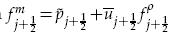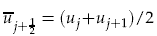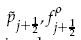Home

# Kinetic Energy Preserving and Entropy Stable Finite Volume Schemes for Compressible Euler and Navier-Stokes Equations

## Abstract

Centered numerical fluxes can be constructed for compressible Euler equations which preserve kinetic energy in the semi-discrete finite volume scheme. The essential feature is that the momentum flux should be of the formwhereandare any consistent approximations to the pressure and the mass flux. This scheme thus leaves most terms in the numerical flux unspecified and various authors have used simple averaging. Here we enforce approximate or exact entropy consistency which leads to a unique choice of all the terms in the numerical fluxes. As a consequence novel entropy conservative flux that also preserves kinetic energy for the semi-discrete finite volume scheme has been proposed. These fluxes are centered and some dissipation has to be added if shocks are present or if the mesh is coarse. We construct scalar artificial dissipation terms which are kinetic energy stable and satisfy approximate/exact entropy condition. Secondly, we use entropy-variable based matrix dissipation flux which leads to kinetic energy and entropy stable schemes. These schemes are shown to be free of entropy violating solutions unlike the original Roe scheme. For hypersonic flows a blended scheme is proposed which gives carbuncle free solutions for blunt body flows. Numerical results for Euler and Navier-Stokes equations are presented to demonstrate the performance of the different schemes.

## Corresponding author

*Corresponding author.Email:praveen@math.tifrbng.res.in

Hide All

# Kinetic Energy Preserving and Entropy Stable Finite Volume Schemes for Compressible Euler and Navier-Stokes Equations

## Metrics

### Full text viewsFull text views reflects the number of PDF downloads, PDFs sent to Google Drive, Dropbox and Kindle and HTML full text views.

Total number of HTML views: 0
Total number of PDF views: 0 *Loading metrics...

### Abstract viewsAbstract views reflect the number of visits to the article landing page.

Total abstract views: 0 *Loading metrics...

* Views captured on Cambridge Core between <date>. This data will be updated every 24 hours.

Usage data cannot currently be displayed.